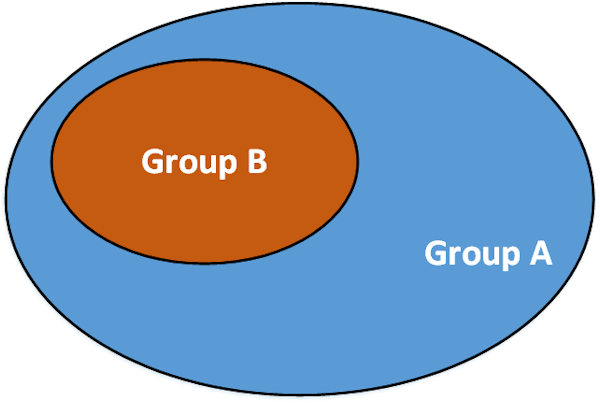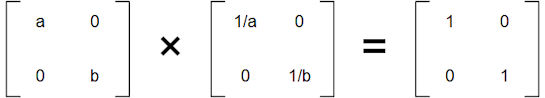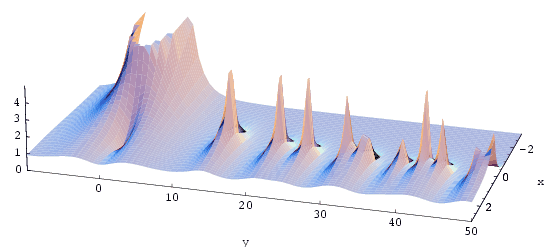# 8 – Simplicity

 < ρℝεν | ℂσητεητs | ℕεχτ >“In character, in manner, in style, in all things, the supreme excellence is simplicity.” – Henry Wadsworth Longfellow

This Chapter, and the two that follow it, may seem at first to be the exception to my rule of sticking only to the Mathematics which advances our goal of understanding the Groups underpinning the Standard Model of Particle Physics. However, not only does this section contain some fundamental elements of Group Theory, which I would be remiss to omit, it also establishes some new Mathematical apparatus, which we will employ in the journey towards our eventual goal.

As flagged in the Foreword, this section features some undergraduate Mathematics, albeit probably only first year. However the majority of the content is less complicated than this and I have tried to place more challenging pieces of logic in boxes which may be read or skipped at the reader’s discretion.

Nevertheless there is a lot of ground to cover and the themes that I want to propound in this part of the book span three Chapters: Simplicity, Normality and Profundity. There is an intentional echo of the French Revolution here as – in Chapter 12 – we will later meet the Father of Group Theory, Évariste Galois, a tragic figure who was the very definition of a Revolutionary Frenchman.

Subsets and SubgroupsIn Chapter 2 I introduced the concept of a sub-set, a set wholly contained in another set, and the symbol used to denote this, ⊂. Specifically we say of two sets A and B:

B ⊂ A

if every element of B is also an element of A 

In Chapter 3 I also briefly noted that Groups could have Subgroups, a Group wholly contained in another Group. The example I cited was that the Cyclic Group of order 3 (or the rotations of an equilateral triangle) was a Subgroup of the Dihedral Group of degree 3 (or the rotations and reflections of an equilateral triangle).

We use a plain old less than sign to state that something is a Subgroup. So if H is a Subgroup of G, we write:

H < G

The formal definition of a Subgroup is as follows:

Given a Group (G,*) , a set H ⊂ G is a Subgroup (H,*) if the following holds:

1. For every a and b ∈ H, a * b ∈ H

2. For every a ∈ H, a-1 ∈ H

The first condition ensures that H is closed. The second that it contains Inverses. A combination of both gives us an Identity as a * a-1 is combining two elements of H and by definition this yields the Identity, which in turn must be part of H by the first condition. Associativity is inherited from G.

Most of the Groups that we have come across in this book have Subgroups, some have infinitely many. If we look at the integers, ℤ, under addition, then each of these is a Subgroup:

2ℤ = {2n where n ∈ ℤ} = {… , -4, -2, 0, 2, 4, …}

3ℤ = {3n where n ∈ ℤ} = {… , -6, -3, 0, 3, 6, …}

4ℤ = {4n where n ∈ ℤ} = {… , -8, -4, 0, 4, 8, …}

and so on…

To see this, consider just the final example (the argument will be the same for the others) and apply our rule from above, we see that:

1. For any two integers, a and b ∈ 4ℤ by definition we can find two other integers x and y such that:

a = 4x

b = 4y

Then

a + b = 4x + 4y = 4(x + y)

Which is clearly a member of 4ℤ, so the first condition holds

2. For any a ∈ 4ℤ let’s again write this as 4x for some integer x. Then

4x + (-4x) = 0

So -4x is the inverse, which is clearly also a member of 4ℤ. So the second condition is also fulfilled

We will refer to these Subgroups of ℤ again in Chapter 9 when we talk about cosets and Quotient Groups.

For a second example, if we consider the multiplication of 2 × 2 matrices with entries in ℝ, then one Subgroup would consist of all diagonal matrices, i.e. ones of the form:Where a and b ∈ ℝ. Again let’s look at our two conditions:

1. If we multiply any two elements together as follows:Then the result is still a diagonal matrix, so condition one holds.

2. It is also simple to construct the inverse of a diagonal matrix as follows:And it is readily seen that this is another diagonal matrix, so our second condition holds.

In Chapter 3 we met the Permutation Groups, Sn. These are Groups relating to the ways in which a set of n elements can be rearranged. If we consider S4, it is fairly evident that S3 is a Subgroup of this. We can create this by keeping one of the four elements to be rearranged in S4 constant and letting only the remaining three be permuted.

Exceptions to the RuleHowever not all Groups have Subgroups. In Chapter 2 we met the Groups ℤn which relate to additional Modulo n (what is the remainder when we divide the result of the addition by n?). In Chapter 3 we saw that these were isomorphic to the Cyclic Groups of the same order (the Group of rotations of an n-sided polygon). Some of these Groups have Subgroups, but others do not. For example:

4 = {0, 1, 2, 3} mod 4

includes the Subgroup {0, 2} mod 4

but

5 = {0, 1, 2, 3, 4} mod 5

has no Subgroups

Using our isomorphic property we can also deduce that C4 has a Subgroup of size 2 (which indeed it does – the 0° rotation and the 180° rotation, omitting the 90° and 270° rotations) but that C5 has no Subgroups.

In general we say that a Group that has no Subgroups is a Simple Group (despite the fact that they may in fact be rather complicated) .

Returning to our ℤn example and noting that the size of ℤ4 is 4 and the size of its Subgroup is 2, we may ponder whether the fact that 4 is divisible by 2 has any relevance here. Further pursuing this line of enquiry, 5 is a prime number and so not divisible by any whole numbers apart from 1 and itself. Does this have any bearing on ℤ5 having no Subgroup?

I won’t work through the proof here but it can indeed be established that:

If n is a prime number then ℤn is a Simple Group

and

If ℤn is a Simple Group, then n must be a prime number 

So we have established at least one connection between Group Theory and the properties of prime numbers. Let’s spend a bit of time talking about the latter.

Primed for ActionAs is inevitable in any field of Mathematics, we have now stumbled upon the prime numbers. I jumped in to using them above with only a passing definition. Here is a slightly more formal one. A prime number is any Natural Number p (p ∈ ℕ) which has no Natural Number divisors except 1 and itself. The first ten primes are:

2, 3, 5, 7, 11, 13, 17, 19, 23, 29…

NB 1 is not normally viewed as being a prime number

At the time of writing, the largest known prime number is:

274,207,281 – 1 

Thousands of years ago Euclid offered an elegant proof that there are infinitely many primes, so this is the largest number we have found to be prime so far, there is no actual largest prime number, they go on for ever.

The Fundamental Theorem of Arithmetic states that any Natural Number can be expressed as a unique product of prime numbers. So we have:

42 = 2 × 3 × 7

128 = 2 × 2 × 2 × 2 × 2 × 2 × 2 = 27

249,999,500 = 24 × 53 × 31 × 1272

Or more generically:

n = p1a1 × p2a2 × … × pmam

Where pi are prime numbers and aj are Natural Numbers

When we say a unique product we ignore doing the multiplications in a different order, 5 × 3 is viewed as the same prime decomposition as 3 × 5.

Again I’m not going to prove this rigorously, but at least part of the argument is easy to grasp and takes the form of a simple set of instructions (the sort of thing that computers are good at doing). One motivation for laying out this argument is that we’ll need to do something similar with Groups and Subgroups later.

1. Is n a prime number?

2. If yes, then write it down in the list of prime factors of n and we are finished

3. If not, then by definition we can find m ∈ ℕ (with m > 1) such that m divides n (with no remainder)

4. Is m a prime number?

5. If so, then write m down in the list of prime factors of n and go back to step 1 but replacing n with n / m

6. If not then by the same arguments as above we can keep looking for factors until one is prime, call this q, write q down in list of prime factors of n and go back to step 1 replacing n with n / q

The above algorithm will generate a list of prime numbers which when multiplied together yields the desired result of n. So we now know that prime factorisations exist. The other requirement is the this list is unique. The general idea behind proving this second part is as follows:

To the contrary assume that there exists four distinct primes, p, q, r and s such that:

pq = rs

Then

p = rs / q

As p is a Natural Number this must mean that q divides the product rs (with no remainder). In antiquity Euclid showed that if a prime number divides the product of two integers, say ab, then it divides a, it divides b or it divides both. Applying this finding to q and rs. Unless q is the same as r, it can’t divide r as r is prime. Similarly q can only divide s if it equals s. So q must equal one or other of r and s, which in turn implies that p must equal the other of r and s.

So, contrary to what we assumed, the two prime factorisations pq and rs are actually identical.

The above result can be extended to establish the general result that prime factorisations are unique, which yields the Fundamental Theorem of Arithmetic.

This observation about the role of the primes in building all numbers is somewhat analogous to how different elements of the Periodic Table combine to create all of the molecules (and macrostructures like humans) that we are familiar with. Perhaps given the subject of this book, primes could be viewed more as being like quarks, the most fundamental building block we have yet to discover (until String Theory gets it act together that is).

As we have seen a number of times through the course of this book, Mathematicians love to generalise previous results. Is there some analogue of the Fundamental Theorem of Arithmetic which holds for Groups and Subgroups. The answer is a definite yes. However before we get there we need to introduce two more concepts. Normal Subgroups and what we meant by decomposing a Group. In the next Chapter we will look at the first of these.

 Concepts Introduced in this Chapter Subgroup A formal definition of the concept introduced in Chapter 3. Simple Group The informal definition is of a Group with no Subgroups. A formal definition is provided in Chapter 9. Prime Numbers Natural Numbers with no Natural Number divisors save for themselves and 1. The Fundamental Theorem of Arithmetic That any Natural Number can be expressed as a unique product of prime numbers (allowing for the ordering of these to vary). Prime Decomposition Algorithm A programmatic way to discover the decomposition of a Natural Number into primes.
 Groups Discovered in this Chapter Diagonal Matrices Square matrices with entries (in ℝ, or indeed ℚ) along just the top left to bottom right diagonal.
 < ρℝεν | ℂσητεητs | ℕεχτ >

Chapter 8 – Notes

  To be 100% accurate here, the symbol I am using also implies that there are elements of A which are not in B, so that B is a proper subset.  Where * is the binary operator. In practice this is often omitted and plain old multiplicative notation is employed instead. So rather than a * b, Mathematicians will write ab. I’ll often use this approach going forward as well, but it is important to note that “multiplication” may not mean multiplication, but some other binary operator.  To be 100% accurate here, a Simple Group is one with no Normal proper Subgroups (where proper has the same meaning as for sets, i.e neither the identity element Subgroup, nor the Group as a whole). As we haven’t yet met Normal Subgroups, I’ll let this definition stand for the present.  Mathematicians save ink by using the formulation “if and only if” to cover this two way arrangement. Being obsessed about economy of expression, this is generally contracted to “iff” – yes that is “if” but with two “f”s. The following symbol is also used to mean the same thing ⇔.  There are infinitely many primes of the type 2n -1, they are called Mersenne Primes after Marin Mersenne a French Mathematician. Smaller examples are 3, 7 and 31. However not all numbers of this format are prime, 24 – 1 = 15 and 15 is clearly 5 × 3. Also there are infinitely many primes which are not equal to 2n – 1 for any n. For example – again at the time of writing – the twelfth largest known prime is not a Mersenne Prime, but instead equal to 19,249 × 213,018,586 + 1. 19,249 is probably as big a number as most people would want to prove was prime without recourse to a computer.
 Text: © Peter James Thomas 2016-18. Images: © Peter James Thomas 2016-17, unless stated otherwise. Published under a Creative Commons Attribution 4.0 International License.